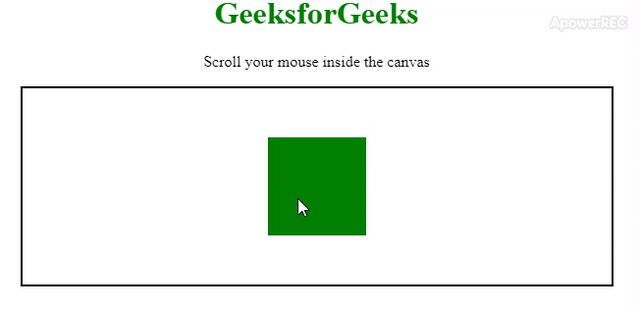GeeksforGeeks App
Open AppBrowser
Continue

# How to zoom in on a point using scale and translate ?

You are given a zoom in to particular image by using scale and translate method. In this zooming process, an image should be zoom in from the center of the image. That image has some width and height in case of rectangular shape and has proper dimensions according to the shape of the image. In zoom in process an image get bigger in size as per requirement. In an image viewer this zoom in process is very important. To get this process you can use scale() and translate() method.

The scale() method scales the current drawing into a smaller or larger size. If you scale a canvas, all future drawings will also be scaled. The position will also be scaled. If you scale(2, 2) drawing will be positioned twice as far from the left and top of the canvas as you specify. The translate() method remaps the (0, 0) position as the canvas. If you have an image and scale it by a factor of 2, the bottom-right point will double in both x and y direction as (0, 0) is the top-left of the image. If you like to zoom the image of the center then a solution is as follows:

• Translate the image.
• Scale the image by x and y factors.
• Translate the image back.

Below example illustrate the above approach:
Example:

 ```<``html``>`` ` `<``head``>``    ``<``title``>``        ``Zooming process using scale and trsnslate``    ````    ``<``style``>``        ``#canvas {``            ``border: 2px solid black;``        ``}``        ``h1{``            ``color: green;``        ``}``    `````` ` `<``body``>``    ``<``center``>``        ``<``h1``>GeeksforGeeks``        ``<``p``>Scroll your mouse inside the canvas``        ``<``canvas` `id``=``"canvas"` `width``=``"600"` `height``=``"200"``>``    ````    ``<``script``>``        ``var zoomIntensity = 0.1;`` ` `        ``var canvas = document.getElementById("canvas");``        ``var context = canvas.getContext("2d");``        ``var width = 600;``        ``var height = 200;`` ` `        ``var scale = 1;``        ``var orgnx = 0;``        ``var orgny = 0;``        ``var visibleWidth = width;``        ``var visibleHeight = height;`` ` `        ``function draw() {``            ``context.fillStyle = "white";``            ``context.fillRect(orgnx, orgny, 800 / scale, 800 / scale);``            ``context.fillStyle = "green";``            ``context.fillRect(250,50,100,100); ``        ``}``        ``setInterval(draw, 800 / 60);``         ` `        ``// Scroll effect function``        ``canvas.onwheel = function(event) {``            ``event.preventDefault();``            ``var x = event.clientX - canvas.offsetLeft;``            ``var y = event.clientY - canvas.offsetTop;``            ``var scroll = event.deltaY < ``0` `? 1 : -2;`` ` `            ``var ``zoom` `= ``Math``.exp(scroll * zoomIntensity);`` ` `            ``context.translate(orgnx, orgny);`` ` `            ``orgnx ``-``= ``x` `/ (scale * zoom) - x / scale;``            ``orgny ``-``= ``y` `/ (scale * zoom) - y / scale;`` ` `            ``context.scale(zoom, zoom);``            ``context.translate(-orgnx, -orgny);`` ` `            ``// Updating scale and visisble width and height``            ``scale *= zoom;``            ``visibleWidth` `= ``width` `/ scale;``            ``visibleHeight` `= ``height` `/ scale;``        ``}``    `````` ` ``

Output :My Personal Notes arrow_drop_up
Related Tutorials